Rotation Worksheets

Rotation worksheets have numerous practice pages to rotate a point, rotate triangles, quadrilaterals and shapes both clockwise and counterclockwise (anticlockwise). In addition, exercises to write the coordinates of the graphed images (rotated shapes) are given here.

Single turn

Follow the instructions and rotate the shape in the clockwise or counterclockwise direction with a single quarter or half turn and draw it in the space provided.Multi turn

Draw the shapes, following the specifications carefully; turning them half or quarter multiple times in both clockwise and counterclockwise directions.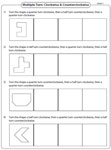Rotation of a point

In these rotation worksheets graph the image of each point according to the given rule. Each worksheet has eight problems for practice.Choose the correct rotation

Rotate the given point about the origin (clockwise or anticlockwise) and choose the correct response from the given multiple choices.Rotation of shapes

Rotate each shape clockwise or counterclockwise about the origin to draw the image of the shape on the given grid.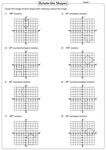Rotation of triangles

Rotate each triangle according to the given instruction. Graph the new position of the rotated triangle.Shapes with four sides and points (quadrilaterals) are shown. Sketch the image of the given shape after rotation.Write the rules

Write a rule to describe each rotation. Mention the degree of rotation (90° or 180°) and the direction of rotation (clockwise or counterclockwise).Write the coordinates: With graph

Rotate each shape. Graph the image obtained and label it. Also write the coordinates of the image. Each worksheet has six problems.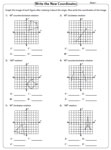Write the coordinates

The coordinates of a figure are given. Make the desired rotation and write down the new coordinates of the rotated image.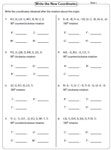Multiple choice: Rotate the shapes

Rotate the given shapes clockwise or anticlockwise and choose the correct image from the multiple choices.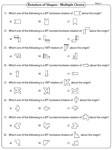The entire collection of worksheets on rotation can be downloaded in a jiffy!

Related Worksheets